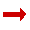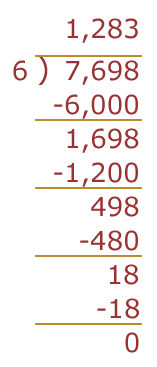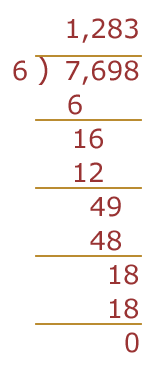# Long Division - Organized Guessing

So, how does Long Division work?

It works by breaking up the big number and solving it a section at a time.

## Thousands, Hundreds, ...

To illustrate, let us try guessing the answer to 7,698 ÷ 6, but following a special method.

We will start at the thousands, then move to the hundreds, then tens, then ones.

OK, starting at the thousands: How many 6 lots of a thousand can we fit into 7,698 ? Well, just one, really. Two is too much:

 1,000 x 6 = 6,000 too small 2,000 x 6 = 12,000 too big

We have made progress of sorts. We can guess that the answer is between 1,000 and 2,000.

Now let's move onto the hundreds. Let's add a hundred at a time:

 1,100 x 6 = 6,600 too small 1,200 x 6 = 7,200 too small 1,300 x 6 = 7,800 too big

So, somewhere between 1,200 and 1,300.

Let's move on to the tens:

 1,210 x 6 = 7,260 too small 1,220 x 6 = 7,320 too small 1,230 x 6 = ...

Stop! All this multiplying is taking too long ...

...there should be an easier way

At the start, when we worked on the thousands, why not chop off what we know already?

We could chop off 1,000 x 6 = 6,000 and get:

 Answer so far Number left 1,000 x 6 = 6,000 7,698 - 6,000 = 1,698

Now (remembering we have chopped off 1,000 x 6) we can continue, but we work on 1,698 now.

 Guesses Compared to 1,698 100 x 6 = 600 too small 200 x 6 = 1,200 too small 300 x 6 = 1,800 too big

Now we can take 200 x 6 = 1,200 from 1,698: 1,698-1200 = 498.

And now we are only working on 498 ...

We can continue like this, following this idea:

Don't work on the entire number every time,
just work on whatever is left.

## Let's try the whole thing from the start, working neatly:

 Thousands 1,000 x 6 = 6,000 too small 2,000 x 6 = 12,000 too big

So the answer for thousands is 1,000 x 6.

7,698 - 1,000 x 6 = 1,698 left to figure out:

 Hundreds 100 x 6 = 600 too small 200 x 6 = 1,200 too small 300 x 6 = 1,800 too big

So the answer for hundreds is 200 x 6.

1,698 - 200 x 6 = 1,698 - 1,200 = 498 left to figure out

 Tens 80 x 6 = 480 too small 90 x 6 = 540 too big

So the answer for tens is 80 x 6

498 - 80 x 6 = 498 - 480 = 18 left to figure out

 Ones 3 x 6 = 18 perfect!

So the answer for ones is 3 x 6

Our Answer: 1,000 x 6 + 200 x 6 + 80 x 6 + 3 x 6 = 7,698, and the answer is 1,283 !

Check: 1,283 x 6 = 7,698

But instead of using all those words, we write it down like this:

 What We Did:Should be Written:And that is how you do Long Division.

Now look at this Long Division page.